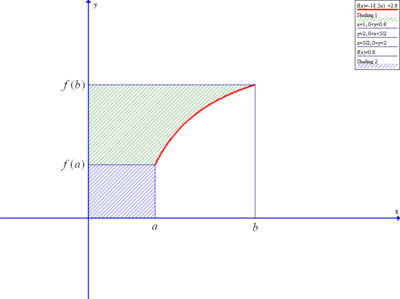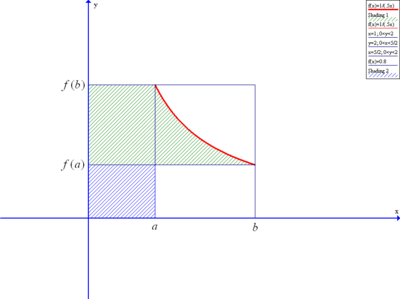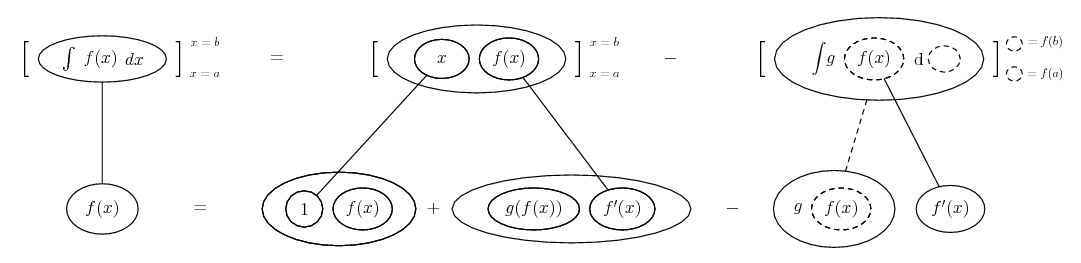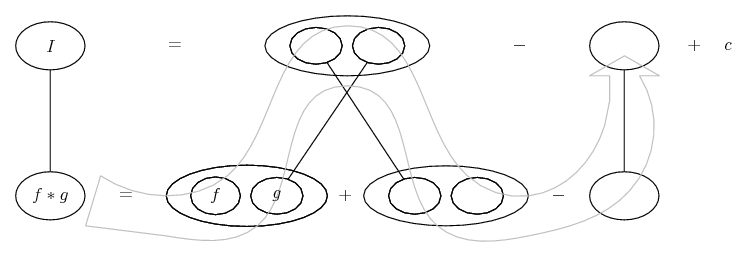# Thread: Proving a formula ..

1. ## Proving a formula ..

Hello

Question:
Prove that if f&g are continous and inverse functions for each other and a&b are constant where b>a .. then :

$\displaystyle \int_a^b f(x) \, dx= b f(b) - a f(a) - \int_{f(a)}^{f(b)} g(x) \, dx$

My FAILED try:

Am thinking about a substitution which makes the f(x) be g(x)
So I substitute x=g(g(x)) ..
But this failed; since dx will be compliacted
Any ideas?

2. Idea: Integration by parts.

3. I think it works for f increasing...... but not decreasing...I.e. subtracting as directed in your formula leaves the white region(s) inside the larger rectangle - which correspond(s) to

$\displaystyle \int_a^b f(x)\ \mathrm{d}x\$ in the increasing case only.

Edit: on the other hand...

Ah! Should have tried integration by parts before sounding off...

Spoiler:Key in next spoiler...
Spoiler:... is the chain rule. Straight continuous lines differentiate downwards (integrate up) with respect to x, and the straight dashed line similarly but with respect to the dashed balloon expression (the inner function of the composite which is subject to the chain rule).... is lazy integration by parts, doing without u and v, and filling out... the product rule from the bottom left corner instead of, as would happen in differentiation, the top one.

Still bothered by my graphs, though...

Late edit: Thanks for exuming this, BayernMunich, but the less said the better! I did spot my VERY SILLY graph error in the end!

4.Originally Posted by BayernMunichHello

Question:
Prove that if f&g are continous and inverse functions for each other and a&b are constant where b>a .. then :

$\displaystyle \int_a^b f(x) \, dx= b f(b) - a f(a) - \int_{f(a)}^{f(b)} g(x) \, dx$

My FAILED try:

Am thinking about a substitution which makes the f(x) be g(x)
So I substitute x=g(g(x)) ..
But this failed; since dx will be compliacted
Any ideas?
Well, come to think of it, f(x) and g(x)--as they are written--cannot, strictly speaking, be inverse functions of each other because they both have the same argument x. In order for two functions to be inverses of each other the one must be the argument of the other and vice versa. I would suggest writing the two functions as y(x) and x(y) and see if that helps to keep track of things.

5. tom@ballooncalculus :
No. It works for both increasing and decreasing functions.
Integrate it by parts with dv=dx and u=f(x) then substitute y=f(x), then the formula will be proved.

6.Originally Posted by Generaltom@ballooncalculus :
No. It works for both increasing and decreasing functions.
Integrate it by parts with dv=dx and u=f(x) then substitute y=f(x), then the formula will be proved.
Yes! As I say, if I'd tried integration by parts in the first place I wouldn't have had any doubt. E.g., see the pic in the spoiler, above. (Works for me!)

So what's wrong with my second graph, I still wonder.

7. Thanks.

#### Search Tags

formula, proving